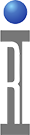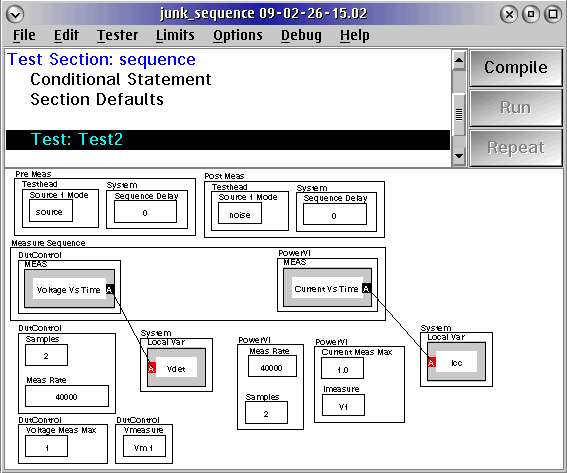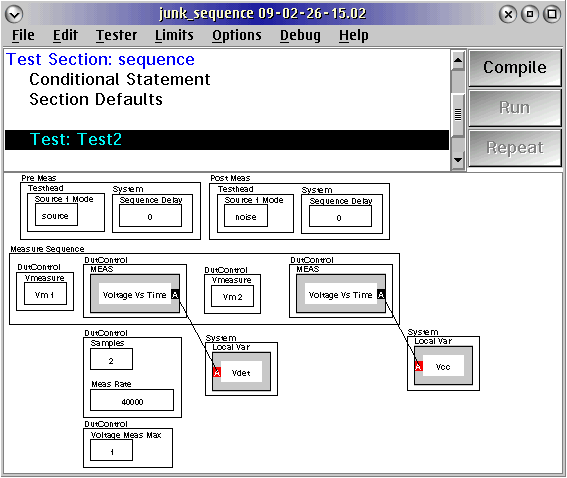Purpose: To create a Measure Sequence group which will force the order in which measurements

are done. This is especially useful when you want to use multiple measurements with a
single setup in a pre-measure group. Without a sequence group, the pre-measure will go
first followed by a measurement, and then the pre-measure group will go again before
another measurement follows. Using a Measure Sequence group will allow the pre-measure
to be done once, followed by the measurements configured in the group.

The following example shows the source being pulsed on followed by two measurements
vs. time (2 samples over 50usec each), and then finished by pulsing the source off. This allows a user to trigger two measurements within an allotted burst. The measure sequence group is similar to that of a pre-measure group in that the buttons are done left to right. The voltage vs. time (Vdet) will measure first over a 50 usec span followed by the current vs. time measurement (Icc), also over a 50usec span.Measurement setup buttons can also be included in the sequence group if needing to use the same
measurement, but for different resources. For example, if you were doing multiple VM measurements,
then you could put Vmeasure: "VM1" then "voltage vs time", followed by "VM2" then "voltage vs time",
etc., all in the Measure Sequence group. It will setup the resources and do the measurements left to right.
An example is shown below:https://roos.com/docs/JLUT-7PW2ZH
ROOS INSTRUMENTS CONFIDENTIAL AND PROPRIETARY